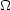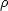### 1. INTRODUCTION

The hot Big Bang theory is the current paradigm for the formation and evolution of the Universe. Parts of the theory that describe the Universe in the first small fraction of a second, like superstring theory, cannot rigorously be tested by experiment and so are not well-constrained at present. But the parts that describe the subsequent evolution of the Universe have been very successful. Examples of the successes are the predictions of the cosmic microwave background (CMB), the abundances of light elements and the expansion of the Universe.

One feature of the model is the partitioning of mass-energy into entities that obey different equations of state. In Table 1, we list current estimates for the densities of these forms of mass-energy ([Fukugita & Peebles 2004], [Allen et al. 2004]) These numbers come from matching the theory to observations of the CMB (e.g. [Spergel et al. 2003]), the distance-redshift relation obtained from Type Ia supernova (e.g. [Riess et al. 2004]), and observations of rich galaxy clusters a different redshifts ([Allen et al. 2004]). The two main components are a dark energy component with density, which could be a cosmological constant 2, and a matter component with densityM. The matter density can further be divided into a dark-matter component and a baryonic component. Inferences about the density of the baryonic component come from observations of the CMB angular power spectrum [Spergel et al. 2003] and the abundances of light elements D, 3He, 4He and 7Li ([Coc et al. 2004]) when compared with big bang nucleosynthesis theory (BBN). The existence of the dark matter component is strengthened by independent evidence from the dynamics and clustering of galaxies and clusters of galaxies (e.g. [de Blok et al. 2001], [Kleyna et al. 2001] and [Borriello & Salucci 2001]). The baryonic component is the subject of the present paper.

 Total density Dark energy density= 1= 0.7 Matter density Dark matter density= 0.3dm = 0.27 Baryonic densityb = 0.03

Understanding the present distribution of material in the Universe is a formidable challenge. With the advent of fast computers and efficient algorithms for calculating the force between many particles, we can now make solid predictions for the current distribution of dark matter ([Bertschinger 1998]). However, understanding the distribution of stars and gas is more difficult. This involves a detailed understanding of the physics underlying galaxy formation: gas hydrodynamics, star formation, and feedback from exploding stars and forming black holes. Despite these difficulties, much progress has been made and simulations are now on the verge of being able to make predictions about where and in what form we should expect to find baryons in the universe today [Mayer 2004]. The new hydrodynamic simulations of galaxy formation (e.g. [Nagamine et al. 2005]) include an intergalactic medium, which makes it straightforward to include these effects.

Technological advances in observational astronomy have also been rapid, mainly as regards our ability to compile and process large datasets. The results from many surveys have recently been published. These include optical redshift surveys, like the Sloan Digital Sky Survey (SDSS; [Abazajian et al. 2004]) and the 2DF Galaxy Redshift Survey [Colless et al. 2001], near-infrared photometric surveys like 2MASS ([Gao et al. 2004]), and HI atomic gas surveys like HIPASS ([Koribalski et al. 2004]).

The time, therefore, is ripe to use the survey results in conjunction with each other to calculate precisely the distribution of baryons in the Universe. A first attempt at such a calculation was made by [Salucci & Persic 1999] for disc galaxies while [Bell et al. 2003] recently presented the baryonic mass function of all galaxies calculated, using a combination of 2MASS and SDSS data. Other authors have computed the stellar mass function of galaxies ([Cole et al. 2001] and [Panter et al. 2004]) or the gas mass function ([Keres et al. 2003], [Koribalski et al. 2004], [Springob 2004]).

In this paper we present the baryonic mass function for nearby galaxies separated by Hubble Type 3. We convert the the field galaxy luminosity function of [Trentham et al. 2005] (which depends on SDSS measurements at the bright end) to a mass function using dynamical mass estimates where possible (e.g. [Kronawitter et al. 2000]) and stellar population synthesis models otherwise (e.g. [Bruzual & Charlot 2003]). We use data from the HIPASS survey ([Koribalski et al. 2004]) to determine the HI (atomic hydrogen) gas masses of galaxies and data from the FCRAO Extragalactic CO survey of [Young et al. (1995]) to determine the H2 (molecular hydrogen) gas masses of galaxies of a given luminosity and Hubble Type. We further use recent X-ray surveys of disc and elliptical galaxies to constrain the contribution of ionised hydrogen [Mathews & Brighenti 2003] and use microlensing data to constrain the contribution of stellar remnants ([Alcock et al. 2000], [Derue et al. 2001] and [Afonso et al. 2003]). Combining all these measurements gives the baryonic mass function.

This paper is organised as follows. In section 2 we present the luminosity function of field galaxies separated by Hubble Type. In section 3 we describe our method for calculating mass-to-light ratios of stellar populations in different galaxies and compute the stellar mass function of galaxies. In section 4 we compute the gas mass function for field galaxies. In section 5 we present the full baryonic mass function of galaxies and compare this with results from the literature. We then discuss baryonic mass components which have been left out of the analysis: halo stars, stellar remnants, and hot ionised gas and dust. In section 6 we integrate the baryonic mass function to obtain the relative contribution of each baryonic mass component tob, gal and compare our results to values ofb derived from the CMB and light element abundances. Finally, in section 7 we present our conclusions.

2 The energy density of a given component,i =i /c, is defined as the ratio of its density to the critical density which would make the universe spatially flat:c = 3H02 / 8G, where G is the gravitational constant and H0 is the Hubble constant at the present epoch, which we assume to be H0 = 70 km s-1Mpc-1. The astronomical unit of a parsec (pc) is 3.09 × 1016 m. Back.

3 The Hubble `tuning fork' galaxy classification scheme separates galaxies by morphology into `early types' (elliptical and lenticular - E, S0) and `late types' (spirals - Sa, Sb, Sc, Sd, Sm - and irregulars - Irr). Dwarf galaxies are denoted dE (dwarf elliptical) or dIrr (dwarf irregular). In this paper we group the dIrr and Irr galaxies together. Back.